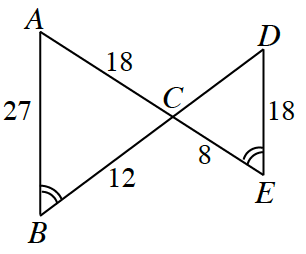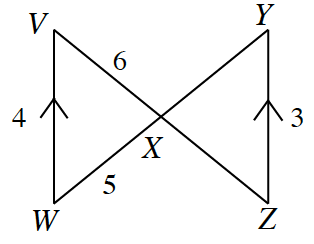### Home > CCG > Chapter 10 > Lesson 10.3.5 > Problem10-182

10-182.

Determine whether or not the two triangles in each pair below are similar. If so, write a flowchart to show your reasoning. If not, explain why not.

1.Similar

This flowchart has 3 bubbles. The top two bubbles have arrows pointing down, to bottom bubble.
$\begin{array}{c} {\enclose{circle}{\; \\ \quad \angle VWX \cong \angle ZYX \quad \\}} \quad {\enclose{circle}{\; \\ \quad \angle WVX \cong \angle YZK \quad \\}} \\ {\Huge \searrow} \qquad {\huge \swarrow} \\ {\enclose{circle}{\; \\ \quad \angle VWX \cong \angle ZYX \quad \\}} \\ \qquad \qquad \qquad \qquad \text{AA} \sim \end{array}$

1.